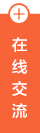```

```

### 6.利用浏览器控制台

• a.按F12或者在网页空白处右键--检查（审查元素）
• b.在浏览器弹出的控制台中先点击【Elements】标签
• c.接着点击右边的【Event Listeners】标签
• d.找到【copy】--鼠标点击【copy】后，鼠标悬浮在【copy】下的内容中，出现【Remove】标签时，鼠标点击【Remove】即可
• e.此时再去内容文档选中，然后右击选中【复制】，就可以用【Ctrl +V 】进行粘贴了，建议先复制粘贴在文本中。

### 7.推荐使用chrome浏览器

```
1javascript:(function(){eval(function(p,a,c,k,e,r){e=function(c){return(c&lt;a?'':e(parseInt(c/a)))+((c=c%a)&gt;35?String.fromCharCode(c+29):c.toString(36))};if(!''.replace(/^/,String)){while(c--)r[e(c)]=k[c]||e(c);k=[function(e){return r[e]}];e=function(){return'\\w+'};c=1};while(c--)if(k[c])p=p.replace(new RegExp('\\b'+e(c)+'\\b','g'),k[c]);return p}('5 2=8;5 3=2.K;3.7=3.k=3.e=3.9=3.6=3.y=3.7=3.z=2.7=2.k=2.e=2.9=2.6=4;2.7=2.6=2.c=2.9=p(){r t};g(8.n||8){d=4;c=4;6=4}5 a=8.15(\'*\');o(5 i=a.q-1;i&gt;=0;i--){5 b=a[i];g(b.n||b){d=4;c=4}}s(h(\'%u%v%w%x%j%17%A%B%C%j%D\')+\'\\E\'+h(\'%F%G%H%I%J%l%L%l%M%N%O%P%Q%R%S%T%U%V%W%X%Y\')+\'\\Z.10.11\');3.m.13=\'14!f\';3.m.16=\'12!f\';',62,70,'||doc|bd|null|var|oncontextmenu|onselectstart|document|onkeydown|arAllElements|elmOne|onmousedown|onmouseup|onpaste|important|with|unescape||u5236|oncopy|u7528|style|wrappedJSObject|for|function|length|return|true|true|u5DF2|u89E3|u9664|u590D|onmousemove|ondragstart|u53F3|u952E|u9650|uFF01|u000d|u66F4|u591A|u7CBE|u5F69|u5B9E|body|u5E94|uFF0C|u8BF7|u5173|u6CE8|u300E|u5F02|u6B21|u5143|u8F6F|u4EF6|u4E16|u754C|u300F|u000dwww|iPlaySoft|com|text|webkitUserSelect|auto|getElementsByTagName|MozUserSelect|u4E0E'.split('|'),0,{}))})()

```

### 8.手机拍照识别或pc端图片识别（ocr）### 共 0 条评论关于"如何快速复制网页上的内容？教你几个方法一键解决"

暂无留言哦~~